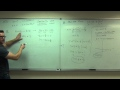Course

Calculus 1 by Leonard

4.62

(58)

Certificate

This course is free today and always will be, from start to finish you will pay nothing.Teacher

Leonard

This Channel is dedicated to quality mathematics education. It is absolutely FREE so Enjoy! Videos are organized in playlists and are course specific.

Course content preview

0h48m

1h37m

1h20m

0h15m

1h27m

3h00m

1h26m

1h50m

1h16m

1h12m

1h02m

0h40m

0h48m

1h34m

1h08m

0h53m

1h34m

0h06m

0h26m

Calculus 1 Lecture 3.4: The Second Derivative Test for Concavity of Functions

0h36mWatch the 1st video of the course

Do not worry, it is free!

Course content0h48m

Calculus 1 Lecture 0.1: Lines, Angle of Inclination, and the Distance Formula

Calculus 1 Lecture 0.1: Lines, Angle of Inclination, and the Distance Formula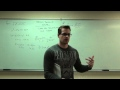1h37m

Calculus 1 Lecture 0.2: Introduction to Functions.

Calculus 1 Lecture 0.2: Introduction to Functions.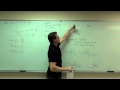1h20m

Calculus 1 Lecture 0.3: Review of Trigonometry and Graphing Trigonometric Functions

Calculus 1 Lecture 0.3: Review of Trigonometry and Graphing Trigonometric Functions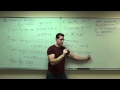0h15m

Calculus 1 Lecture 0.4: Combining and Composition of Functions

Calculus 1 Lecture 0.4: Combining and Composition of Functions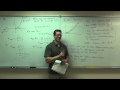1h27m

Calculus 1 Lecture 1.1: An Introduction to Limits

Calculus 1 Lecture 1.1: An Introduction to Limits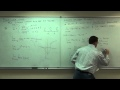3h00m

Calculus 1 Lecture 1.2: Properties of Limits. Techniques of Limit Computation

Calculus 1 Lecture 1.2: Properties of Limits. Techniques of Limit Computation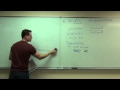1h26m

Calculus 1 Lecture 1.4: Continuity of Functions

Calculus 1 Lecture 1.4: Continuity of Functions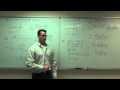1h50m

Calculus 1 Lecture 1.5: Slope of a Curve, Velocity, and Rates of Change

Calculus 1 Lecture 1.5: Slope of a Curve, Velocity, and Rates of Change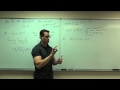1h16m

Calculus 1 Lecture 2.1: Introduction to the Derivative of a Function

Calculus 1 Lecture 2.1: Introduction to the Derivative of a Function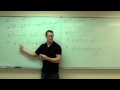1h12m

Calculus 1 Lecture 2.2: Techniques of Differentiation (Finding Derivatives of Functions Easily)

Calculus 1 Lecture 2.2: Techniques of Differentiation (Finding Derivatives of Functions Easily)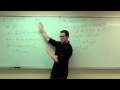1h02m

Calculus 1 Lecture 2.3: The Product and Quotient Rules for Derivatives of Functions

Calculus 1 Lecture 2.3: The Product and Quotient Rules for Derivatives of Functions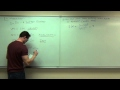0h40m

Calculus 1 Lecture 2.4: Applications of the Derivative

Calculus 1 Lecture 2.4: Applications of the Derivative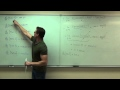0h48m

Calculus 1 Lecture 2.5: Finding Derivatives of Trigonometric Functions

Calculus 1 Lecture 2.5: Finding Derivatives of Trigonometric Functions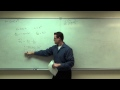1h34m

Calculus 1 Lecture 2.6: Discussion of the Chain Rule for Derivatives of Functions

Calculus 1 Lecture 2.6: Discussion of the Chain Rule for Derivatives of Functions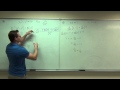1h08m

Calculus 1 Lecture 2.7: Implicit Differentiation

Calculus 1 Lecture 2.7: Implicit Differentiation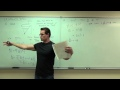0h53m

Calculus 1 Lecture 2.8: Related Rates

Calculus 1 Lecture 2.8: Related Rates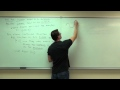1h34m

Calculus 1 Lecture 3.1: Increasing/Decreasing and Concavity of Functions

Calculus 1 Lecture 3.1: Discussion of Increasing and Decreasing Intervals. Discussion of Concavity of functions.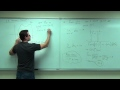0h06m

Calculus 1 Lecture 3.2: A BRIEF Discussion of Rolle's Theorem and Mean-Value Theorem.

Calculus 1 Lecture 3.2: A BRIEF Discussion of Rolle's Theorem and Mean-Value Theorem.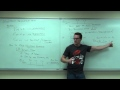0h26m

Calculus 1 Lecture 3.3: The First Derivative Test for Increasing and Decreasing

Calculus 1 Lecture 3.3: The First Derivative Test for Increasing and Decreasing of Functions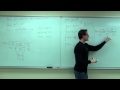0h36m

Calculus 1 Lecture 3.4: The Second Derivative Test for Concavity of Functions

Calculus 1 Lecture 3.4: The Second Derivative Test for Concavity of Functions